cancel
Showing results for
Did you mean:## Unlocking the Power of RANKX: A Deep Dive into DAX Ranking

Consider a function to construct and compute ranks based on the RANKX function.

Consider our data modelTable Orders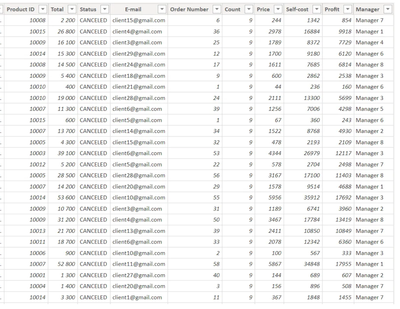We will rank the products by the Total and Self-Cost fields.

Let's build a summary table, which we get from the Orders table using the SUMMARIZE function and sort by descending ProductTotal field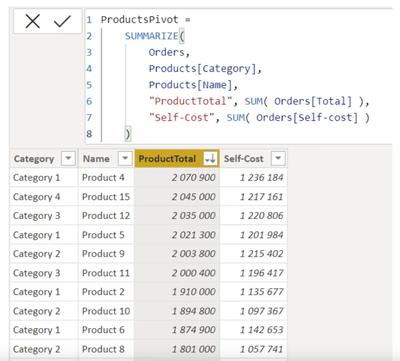Create ranks on the Orders table. The ranks will be created relative to the products in the Total column. Simply put, we need to arrange the products in descending order of their sales amounts. Go to the Report tab, create a measure using the RANKX function and create a summary table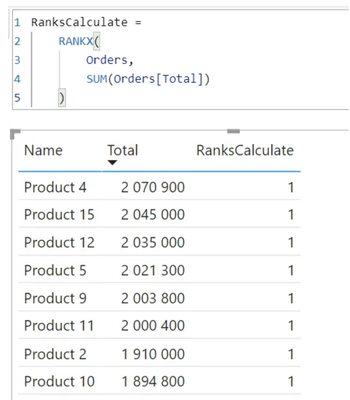Our ranks should be ranked according to the sales amounts spread across each product, but we see that the measure didn't work as it should - rank 1 is returned everywhere. The point is that when DAX calculated this cell, it first evaluated the entire filter context, and the product context is filtered by Product4 for the top row, etc.

To fix the error, we need to remove the previous filters. Here we introduce ALLSELECTED and CALCULATE functions for the Products table, since the Name field is taken from itLet's try to build ranks not by the sum by products, but by the number of orders. Let's display the table with the number of orders by products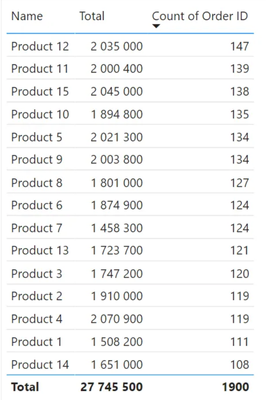To change the rank calculation, you need to change the table code to return unique rows for each product rather than rows for each order.Taking a closer look at the table we can see that the ranks for the individual products are the same. In order to correct the situation we can use the fifth parameter of the RANKX functionLet's complicate our example and calculate Profit in this expression instead of Total. Let's create ranks by products with respect to profit. To calculate the profit, we need to enter the SUMX function.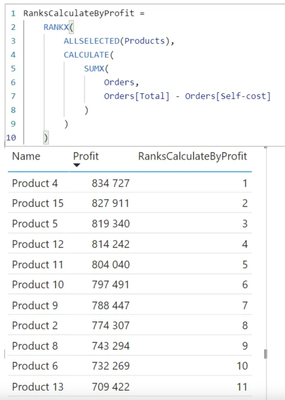Profit is calculated through the calculated columnLet's build another table by product categoryWe have considered the construction of ranks in terms of measures. Let's consider the construction of ranks in computable tables.

There is no external custom filter context in the tables. The external custom filter context does not affect the tables of the stored data model in any way. Therefore, the formula will be slightly different.

Create a new column to calculate ranks relative to the Total column and write the RANKX function.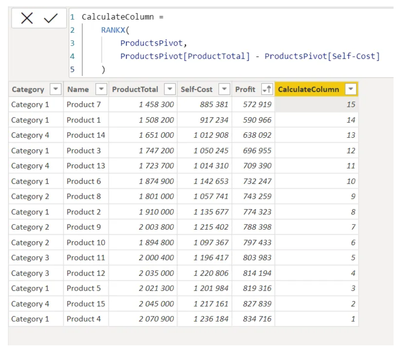The Profit field is calculated through the difference of ProductTotal and Self-Cost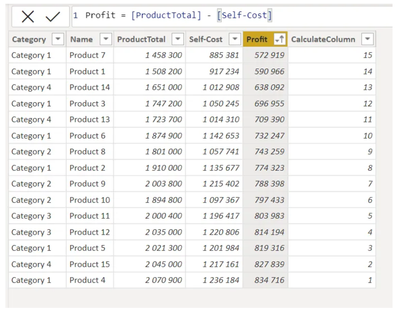Top Kudoed Posts
Latest Articles
Archives
Polls
What is your favorite Power BI feature release for September 2023?